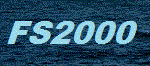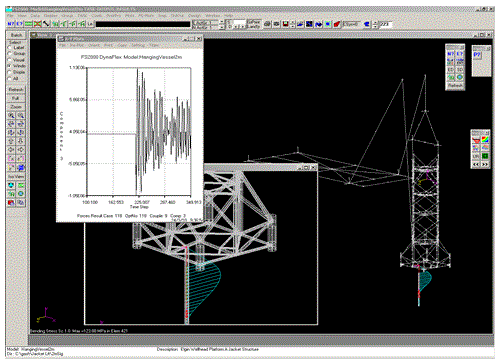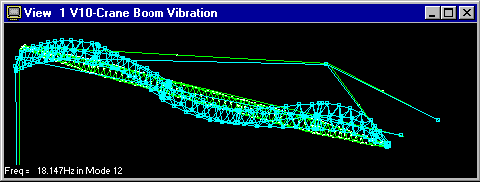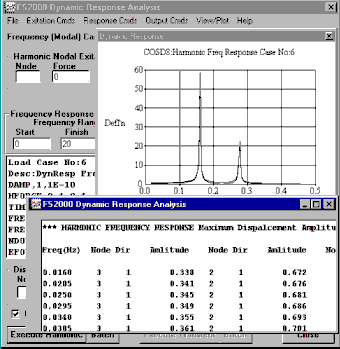﻿ FS2000 Dynamic Analysis Software### FS2000 has three fundamental dynamic analysis capabilities:

• Frequency Analysis - This forms part of the core module of FS2000 and is used to evaluate structural natural frequencies (Vibration modes).

• Dynamic Response (Normal Mode Response) - This is an optional module - FS-Dynamic
Harmonic Response
Transient Response
Spectral Response

• Linear/Non-linear Time History - This an optional module - FS-DyNoFlex
Incremental dynamic solver

#### Frequency Analysis (FS-Frequency)

FS-Dynamic is the dynamic response module of FS2000. The analysis is based on the normal mode method. The Frequency Analysis module of FS2000, which is included in the core module of FS2000 is used to evaluate the frequencies and mode shapes of linear structural models. The response module is used to evaluate the resulting deflections, forces and stresses due to various forms of excitation.

• Load Case/Mass Case Conversion - Standard Load cases can be converted to Mass Definition Cases by using a mass conversion constant.• Multiple Mass Cases - Any number of mass definition cases may be used to create the generated load cases.
• Lumped/Consistent Mass Model
• Jacobi or Subspace Iteration Methods
• Graphical Output - Animated mode shape displays

#### Response Analysis (FS-Dynamic)

• Harmonic Response
• Constant Amplitude - Forces or moments
• Rotating Unbalance
• Support Motion - Displacement, velocity or acceleration
• Frequency Response - Amplitude spectrum input• Deflection/Force- Response plots or tabulated output at selected nodes or elements
• Full Std Result Case Output - Snapshot Output at Specific time and frequency
• Transient Analysis
• Damping - Critical damping ration for each mode
• Initial Conditions - Nonzero initial displacements and velocities
• Time Dependent Excitation - Forces or moments using a defined curve or a generated curve based on a damped harmonic signal.
• Shock/Impact - Excitation due to undamped shock/impact on an undamped system. Standard pulse curves eg triangle, half sine etc.
• Support Excitation - Base displacement using a defined curve or a generated curve based on a damped harmonic signal.
• Deflection/Force- Time history response plots or tabulated output at selected nodes or elements
• Full Std Result Case Output - Snapshot Output at Specific time and frequency
• Response Spectrum Analysis
• Response Spectra Curves - Displacement, velocity or acceleration spectra
• Multiple Mass Cases - Any number of mass definition cases may be used to create the frequency cases. Standard Load cases can be converted to Mass Definition Cases by using a mass conversion constant.